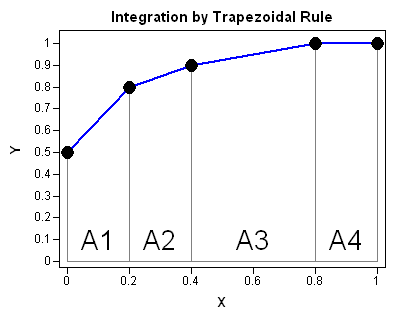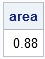In a previous article I discussed the situation where you have a sequence of (x,y) points and you want to find the area under the curve that is defined by those points. I pointed out that usually you need to use statistical modeling before it makes sense to compute the area.

However, there is a numerical technique that is very useful for a wide range of numerical integration scenarios, and that is the trapezoidal rule.

The following graph illustrates the trapezoidal rule. Given a set of points (x1, y1), (x2, y2), ..., (xn, yn), with x1x2 ≤ ... ≤ xn, the trapezoidal rule computes the area of the piecewise linear curve that passes through the points. You can compute the area under the piecewise linear segments by summing the area of the trapezoids A1, A2, A3, and A4. (Sometimes a trapezoid is degenerate and is actually a rectangle or a triangle.)### Implementing the Trapezoidal Rule in SAS/IML Software

It is easy to use SAS/IML software (or the SAS DATA step) to implement the trapezoidal rule. The area of a trapezoid defined by (xi, yi) and (xi+1, yi+1) is

( xi+1 – xi ) ( yi + yi+1 ) / 2
The first term is just the width of the subinterval [xi, xi+1] and the second term is the average of the heights at each end of the subinterval.

The following user-defined function computes the area under the piecewise linear segments that connect the points. The function does not check that the x values are sorted in nondecreasing order.

```proc iml; /**Given two vectors x,y where y=f(x), this module approximates the definite integral int_a^b f(x) dx by the trapezoid rule. The vector x is assumed to be in numerically increasing order so that a=x and b=x[nrow(x)]. The module does not assume equally spaced intervals. The formula is Integral = Sum( (x[i+1] - x[i]) * (y[i] + y[i+1])/2 ) **/ start TrapIntegral(x,y); N = nrow(x); dx = x[2:N] - x[1:N-1]; meanY = ( y[2:N] + y[1:N-1] )/2; return( dx` * meanY ); finish;   /** test it **/ x = {0.0, 0.2, 0.4, 0.8, 1.0}; y = {0.5, 0.8, 0.9, 1.0, 1.0}; area = TrapIntegral(x,y); print area;```Notice that the implementation does not require equally spaced points. The width of all subintervals are computed in a single statement and assigned to the vector dx. Similarly, the average of the heights are computed in a single statement and assigned to the vector meanY. The summation of all the areas is then computed by using a dot product of vectors. (Equivalently, the module could also return the quantity sum(dx # meanY), but the dot product is the more efficient computation.)

The simplicity of the trapezoidal rule makes it an ideal for many numerical integration tasks. Also, the trapezoidal rule is exact for piecewise linear curves such as an ROC curve. Also, as John D. Cook points out, there are other situations in which the trapezoidal rule performs more accurately than other, fancier, integration techniques.

The trapezoidal rule is not as accurate as Simpson's Rule when the underlying function is smooth, because Simpson's rule uses quadratic approximations instead of linear approximations. The formula is usually given in the case of an odd number of equally spaced points. Leave a comment to discuss the relative advantages and disadvantages of Simpson's rule as compared to the trapezoidal rule.

In a future blog post, I will use the TrapIntegral function to integrate some functions that arise in statistical data analysis.

ShareDistinguished Researcher in Computational Statistics

Rick Wicklin, PhD, is a distinguished researcher in computational statistics at SAS and is a principal developer of SAS/IML software. His areas of expertise include computational statistics, simulation, statistical graphics, and modern methods in statistical data analysis. Rick is author of the books Statistical Programming with SAS/IML Software and Simulating Data with SAS.

1.Charlie Huang on

Fantastic work! I applied it in my daily routine immediately. Thanks a lot.

2.If you could state the strengths and limitations of both simpson's rule and trapezodial rule that would be greatly appreciated. However, the work already posted is great.

•The strength of the trapezoidal rule is that it is fast and it is exact for piecewise linear functions. The strength of Simpson's rule is that it is usually more accurate: it has a smaller error when integrating smooth functions. The limitations for both algorithms is that they are designed for finite intervals. They are also require that you choose the number of subdivisions in advance, which is why I use the QUAD subroutine in SAS/IML software for serious work. The QUAD subroutine implements an adaptive Romberg-type algorithm that is is more accurate than Simposon's Rule and can work on infinite domains.

3.I need some help on the application of trapezoidal rule or simpson rule in mining metallurgy, where exactly can we apply these two rules?

•These rules enable you to numerically approximate an integral. Integrals appear in many areas of material science, including loads, shears, stresses, torsion, etc. See any engineering or calculus textbook.

4.what about missing data points? what is the best way to estimate area under the curve if there is a missing data point?

•Use the trapezoid rule applied to the nonmissing data, which is equivalent to linear interpolation of missing Y values.

•So if I have two groups (placebo vs active) and 5 timepoints (i.e., 4 trapezoids) for a measurement scale. Several of the subjects are missing the last 1-2 timepoints. If I sum the values of the trapezoids to a final AUC for each subject, can I simply compare mean AUC's with a t-test (assuming normally distributed)?.

If so, some of the total AUCs will be much smaller because of missing values--how can I handle this post hoc?

•If you want to compare two ROC curves, use the ROC and ROCCONTRAST statements in PROC LOGISTIC. If you have further questions, you can post them to the SAS Support Communities.

5.Hello,
Thanks for your explanation. How can I implement this code in to the Malab script?

6.I want to make a report in Trapezoid rule and simpson rule.
•7.